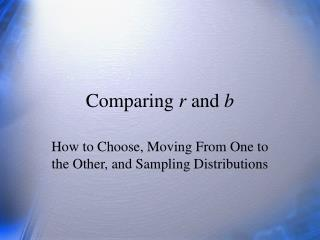DownloadDownload PresentationComparing r and b

# Comparing r and b

Télécharger la présentation## Comparing r and b

- - - - - - - - - - - - - - - - - - - - - - - - - - - E N D - - - - - - - - - - - - - - - - - - - - - - - - - - -
##### Presentation Transcript

1. Comparing r and b How to Choose, Moving From One to the Other, and Sampling Distributions

2. How is the raw score slope related to the correlation coefficient? Describe a concrete situation where two groups have the same correlation between two variables but different slopes. Describe a concrete situation where you would prefer r to b. Describe the sampling distribution of r. Include bias, sampling variance, skew, sample size, power. Draw a picture and describe the sampling distribution of the regression line. Questions

3. Slope Estimates • r is b when X and Y are z scores. • The test for the significance of the difference between groups for r and b means 2 different things. • Males: r = .30, SX=50, SY=1, b=.006. • Females:r = .60, SX=100, SY=1, b=.006. • What if r = .60 for both groups? b = ? With correlation, there is only standardized slope. With regression, there is slope, intercept and standard error of prediction.

4. Choice Between r and b • Always report correlation matrix with M & SD so people can choose. • Correlation to show strength of association between vbls or across settings • Regression for prediction problems • If units have meaning, may want regression (consider slope and intercept), e.g., SAT = 0; change in GPA, graduation rate, etc.

5. Sampling Distribution of r Sampling distribution depends on N and ρ. M=.295, slight bias. Slight negative skew. Big power problem. With N=50, critical value of r is .27, so about half of observed rs will not be significant. Power is about .5. This size correlation and sample are common in psych.

6. Sampling Distribution of r (2) M=.795, slight bias. A little more negative skew. No power problem. Uncommon situation unless you are estimating reliability. Correlation and regression demand large samples for significant results unless the effects of the IV are very large. Large effects are not common in most areas of psychology (social science generally).

7. Empirical Sampling Distributions, rho = .0 to rho = .9

8. Sampling Distribution of Regression Line Note fan shape. You will see this in the line’s confidence interval. The means of X and Y are typically pretty well estimated. The line always goes thru Xbar, Ybar. A little difference in the slope has little impact on the line close to the mean, but more and more the farther out we get from the mean of X. Note the relation to leverage.

9. Review • How is the raw score slope related to the correlation coefficient? • Describe a concrete situation where two groups have the same correlation between two variables but different slopes. • Describe a concrete situation where you would prefer r to b. • Describe the sampling distribution of r. Include bias, sampling variance, skew, sample size, power. • Draw a picture and describe the sampling distribution of the regression line.